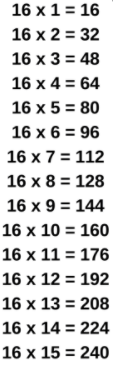# 16 Times Table- Learn Table Of 16 : Multiplication Table Of 16

Safalta expert Published by: Yashaswi More Updated Wed, 08 Jun 2022 10:17 PM IST

## Highlights

Check out how to learn the 16 Times table easily here at Safalta.com

Multiplication is a fundamental operation in mathematics. Learning how to multiply numbers is a useful skill to have. In order to learn more advanced mathematical concepts in school, students must first master the multiplication table 1 – 20. Learning the 16 times table, for example, assists children in solving multiplication and division problems involving multiples of 16.A 16 times table is a multiplication table formed by multiplying the number 16 by various whole numbers. Learning times tables aids in the transition from finger-counting to mental arithmetic.

This mini-lesson contains the 16 times table, which is the multiplication of 16 with various whole numbers, as well as some tricks for memorizing the table.

### Free Demo ClassesSource: Safalta.com

Join Safalta School Online and prepare for Board Exams under the guidance of our expert faculty. Our online school aims to help students prepare for Board Exams by ensuring that students have conceptual clarity in all the subjects and are able to score their maximum in the exams.

### 16 Times Table Chart:## Multiplication Table of 16

• Learning the Multiplication Table of 16 during formative years helps students solve long problems involving multiplication and division.
• 16 times table helps in visualizing the patterns of multiples and applying them in real-world scenarios.

Go through the 16 times table that is given below for fast calculations.

16 Times Table
 16 Times Table up to 10 16 × 1 = 16 16 × 6 = 96 16 × 2 = 32 16 × 7 = 112 16 × 3 = 48 16 × 8 = 128 16 × 4 = 64 16 × 9 = 144 16 × 5 = 80 16 × 10 = 160

## Tips for 16 Times Table

1. 16 doesn’t have any rules that make the multiplication table of 16 easy to memorize, but there is a pattern for every five multiples of sixteen: 16, 32, 48, 64, 80, 96, 112, 128, 144, 160

2. The last digit of these multiples always repeats, which means that students can remember these digits to help them with the 16 times table.

### Table of 16 up to 20

We have obtained the first ten multiples of 16. Let us evaluate the table of 16 up to 20.

## Worksheet on 16 Times Table

1. ### Example 1: Using 16 times table, evaluate 16 times 8 minus 10.

Solution:

First, express 16 times 8 minus 10 mathematically i.e. 16 times 8 minus 10

Using 16 times table, we have 16 times 8 = 128 i.e. 128 - 10 = 118

Hence, 16 times 8 minus 10 is 118.

2. ### Example 2: Solve using multiplication table of 16: 16 times 5 plus 12.

Solution:

First, we will write 16 times 5 plus 12 mathematically.

Using 16 times table, we have, 16 times 5 plus 12 = 16 × 5 + 12 = 80 + 12 = 92

Hence, 16 times 5 plus 12 is 92.

## What is the importance of learning Table 2 to 20?

For making mathematical section easier, memorising table 2 to 20 is important.

## How can I learn tables easily?

Daily recite the table as mentioned in the article twice and thrice.

## What is the 16 times table?

16 Times Table up to 10
16 × 1 = 16 16 × 6 = 96
16 × 2 = 32 16 × 7 = 112
16 × 3 = 48 16 × 8 = 128
16 × 4 = 64 16 × 9 = 144

## What are the factors of 16?

Also 1 and 16 are factors of 16 because 1 x 16 = 16. The factors of 16 are 1, 2, 4, 8, 16. You can also think about factors in terms of division: The factors of a number include all numbers that divide evenly into that number with no remainder.

## What is the multiple of 16?

The multiples of 16 are 16, 32, 48, 64, 80, 96, 112, 128, 144, 160,… Hence the common multiples of 12 and 16 are 48, 96, 144,… and their LCM is 48.